# Collision Model HS1¶

This SIMION user program implements a rather complete hard-sphere collision model. Collision models are useful for simulating non-vacuum conditions, in which case ions collide against a background gas and are deflected randomly.

Features and assumptions of the model:

• Ion collisions follow the hard-sphere collision model. Energy transfers occur solely via these collisions.
• Ion collisions are elastic.
• Background gas is assumed neutral in charge.
• Background gas velocity follows the Maxwell-Boltzmann distribution.
• Background gas mean velocity may be non-zero.
• Kinetically cooling and heating collisions are simulated.
• Background gas as a whole is unaffected by ion collisions.

## Code¶

• SIMION 8 includes a Lua version (collision_hs1 example). It is more updated and documented than earlier PRG/SL versions.

## Evaluation and Comparison¶

The figure below shows ion trajectories in the HS1 collision model (dots mark collision events). Conditions: ions of mass 200 amu, 15 angstrom collision diameter, and initial velocity to the right at 24 eV colliding against a He background gas with 2 angstrom collision diameter, 4 mTorr pressure, and 275 K temperature. Collisions tend to kinetically cool ions initially. As ions slow down, mean-free-path decreases and the scattering effect increases.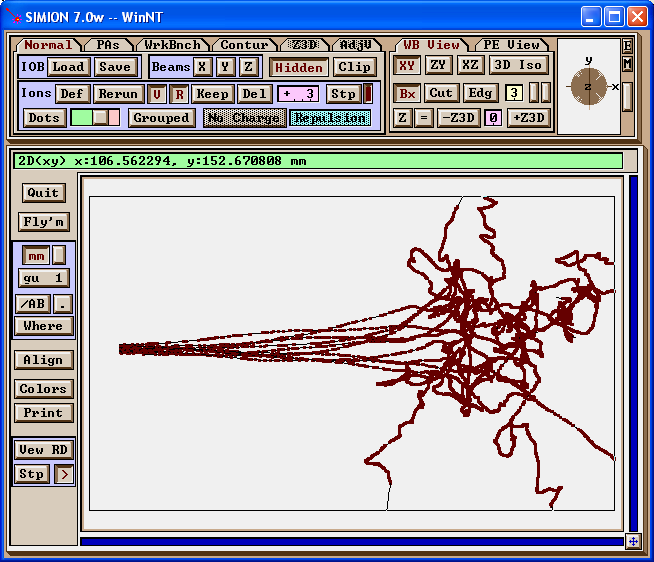The below figures area plots of ion speed per collision number using the HS1 collision model as well as the earlier dahl_drag.prg and _Trap/INJECT.PRG in SIMION 7.0 models for comparison. The Ling1997 paper (Figure 4) provides a similar graph for its collision model under the same conditions. The HS1 and dahl_drag.prg models are in fairly good agreement in this aspect. However, these differ from the Ling1997 and _Trap/INJECT.PRG graphs, which are similar to each other and show almost twice as rapid dampening. (Note: full details of Ling1997 are not available.) The reduced dampening in the former models seems partly due to the inclusion of heating collisions from behind the ions.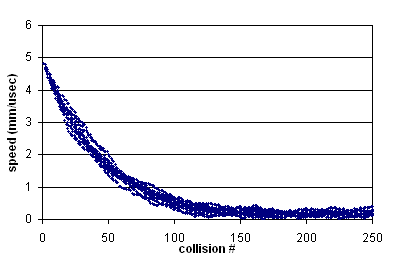Fig. 61 Figure: Dampening using Collision Model HS1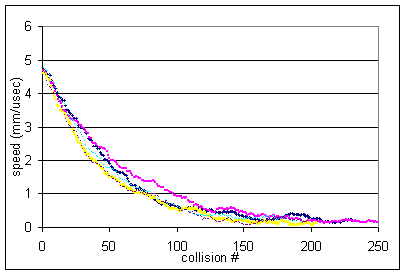Fig. 62 Figure: Dampening using Collision Model dahl_drag.prg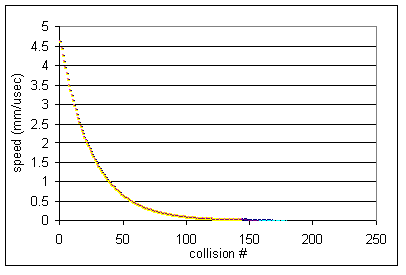Fig. 63 Figure: Dampening using Collision Model _Trap/INJECT.PRG in SIMION 7.0 (also resembles Ling1997)

Despite these similarities in the above regard, the models can still be quite different. For example, HS1 model supports a variable mean-free-path (unlike dahl_drag.prg), and this affects the frequency of collisions, especially as speeds change. Models can also handle angular scattering differently (e.g. _Trap/INJECT.PRG does not provide any angular scattering).

## Appendix: Derivation of Mean Relative Speed¶

This program calculates mean relative speed between the ion and background gas in order to calculate mean-free-path. The following is a derivation of the equation for mean relative velocity.

Compute average relative speed c of a single particle (ion) against a background gas (gas). The background gas is assumed to be Maxwell distributed in velocity.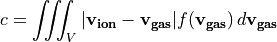where f is the three-dimensional Maxwell distribution given by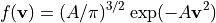such that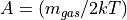.

Substituting,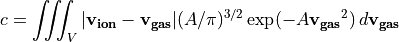Let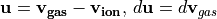.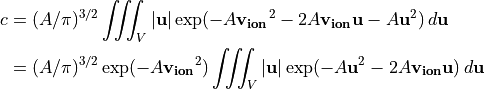Convert to spherical coordinates and let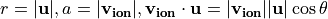.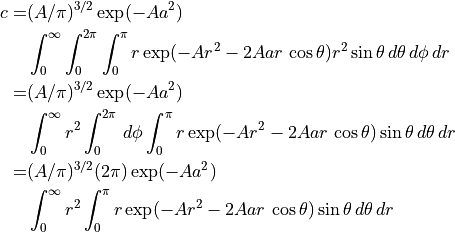Let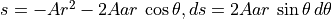.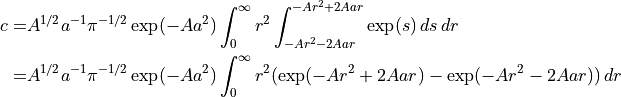To solve this integral, we use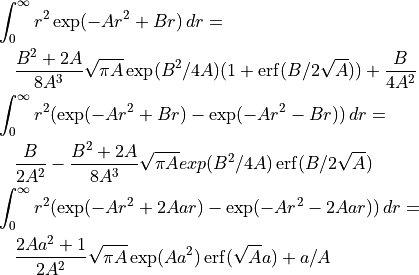Substituting,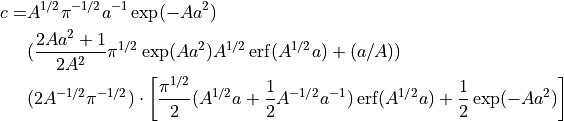Let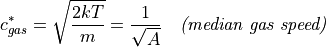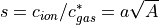Substituting gives the result: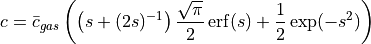This result is in agreement with Ding2002.

Note the following resuts:

As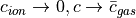.

Also, as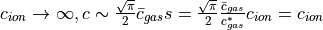.

Further, if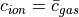, then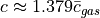, which is approximately the average relative speed between the gas particles themselves (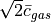).

The above results provide a rough justification for the approximation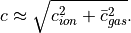.